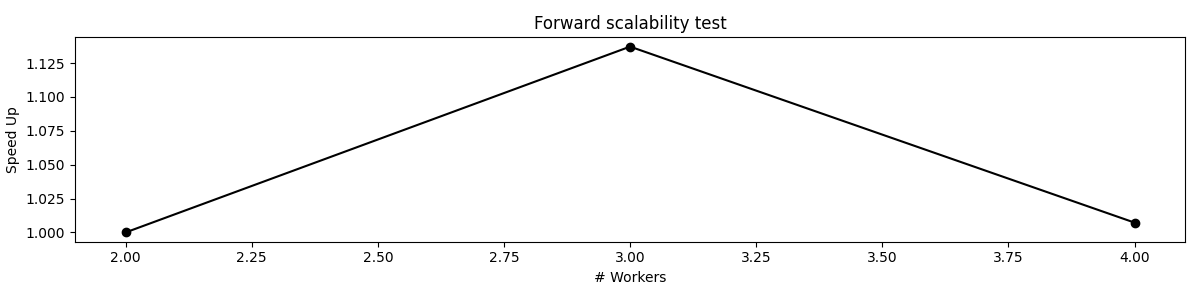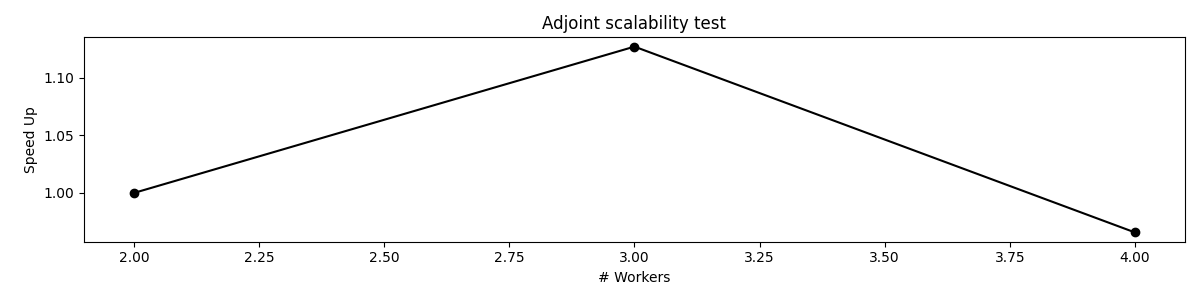# Operators with Multiprocessing#

This example shows how perform a scalability test for one of PyLops operators that uses `multiprocessing` to spawn multiple processes. Operators that support such feature are `pylops.basicoperators.VStack`, `pylops.basicoperators.HStack`, and `pylops.basicoperators.BlockDiagonal`, and `pylops.basicoperators.Block`.

In this example we will consider the BlockDiagonal operator which contains `pylops.basicoperators.MatrixMult` operators along its main diagonal.

```import matplotlib.pyplot as plt
import numpy as np

import pylops

plt.close("all")
```

Let’s start by creating N MatrixMult operators and the BlockDiag operator

```N = 100
Nops = 32
Ops = [pylops.MatrixMult(np.random.normal(0.0, 1.0, (N, N))) for _ in range(Nops)]

Op = pylops.BlockDiag(Ops, nproc=1)
```

We can now perform a scalability test on the forward operation

```workers = [2, 3, 4]
compute_times, speedup = pylops.utils.multiproc.scalability_test(
Op, np.ones(Op.shape), workers=workers, forward=True
)
plt.figure(figsize=(12, 3))
plt.plot(workers, speedup, "ko-")
plt.xlabel("# Workers")
plt.ylabel("Speed Up")
plt.title("Forward scalability test")
plt.tight_layout()
``````Working with 2 workers...
Working with 3 workers...
Working with 4 workers...
```

And likewise on the adjoint operation

```compute_times, speedup = pylops.utils.multiproc.scalability_test(
Op, np.ones(Op.shape), workers=workers, forward=False
)
plt.figure(figsize=(12, 3))
plt.plot(workers, speedup, "ko-")
plt.xlabel("# Workers")
plt.ylabel("Speed Up")```Working with 2 workers...